# Digits

Write the smallest and largest 1-digit number.

Correct result:

min =  1
max =  9

#### Solution:

$min=1$
$max=9$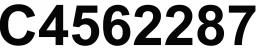We would be very happy if you find an error in the example, spelling mistakes, or inaccuracies, and please send it to us. We thank you!Tips to related online calculators
Would you like to compute count of combinations?

## Next similar math problems:

• Number unknownAdela thought the two-digit number, she added it to its ten times and got 407. What number does she think?
• Mystery numberA mystery number is between 800 and 920. No digits are the same. The difference of the numbers 5. What is the mystery number?
• Roman numerals 2+Add up the number writtens in Roman numerals. Write the results as a roman numbers.
• Roman numeralsWrite numbers written in Roman numerals as decimal.
• Write decimalsWrite in the decimal system the short and advanced form of these numbers: a) four thousand seventy-nine b) five hundred and one thousand six hundred and ten c) nine million twenty-six
• What is missing (1000)What number is to add to get 1000?
• I thinkI think a number. When I multiply it by five, and after that I subtract 477, I get the same number as if I multiplied it twice. What number do I think?
• The number 3Ski organizers should print the start numbers from 1 to 45. How many times will they use the number 3 when printing?
• SummandOne of the summands is 145. The second is 10 more. Determine the sum of the summands.
• ClassmatesRoman is ranked 12th highest and eleventh lowest pupil. How many classmates does Roman have?
• Balls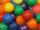I have 19 balls and my friend has 27. About how many has my friend more balls?
• Farm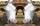Agricultural cooperative (farm) produced 14144 liters of milk for 16 days. Which exceeded the plan by 1984 liters. How many liters of milk a day had planned to produce?
• School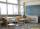27 students of 6.D class is going to a trip. They pay 9 € each. The teacher got 153 €. How many children have not paid?
• To thousands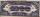Round to thousands following numbers:
• Compare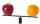Compare with characters >, <, =: 85.57 ? 80.83
• Angles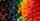Which of those angles are obtuse?
• DoctorsIn the city operates 196 doctors. The city has 134456 citizens. How many citizens are per one doctor?